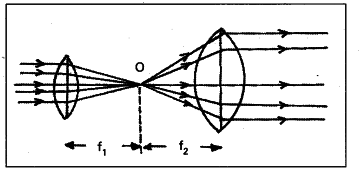# Two converging lenses are to be placed in the path of parallel rays

Two converging lenses are to be placed in the path of parallel rays so that the
rays remain parallel after passing through both lenses. How should the lenses be arranged ? Explain with a neat ray diagram.

i) A parallel beam of light rays will converge on focal point of the lens after refraction.
ii) Light rays passes through the focal point will parallel to principal axis after re-fraction.
iii) So the two lenses are arranged ’ on a common principal axis such that their focal points coincide with each other, then the rays remain parallel after passing through both lenses.